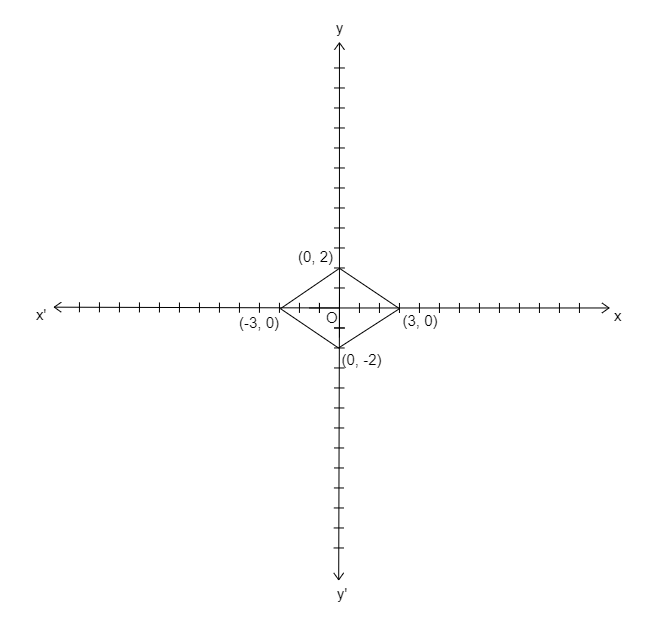# Plot the points $( 0,\ 2),\ ( 3,\ 0),\ ( -2,\ 0)$ and $( 0,\ -3)$ on the graph. Which figure do we obtain if we join the points?

Given: Points $( 0,\ 2),\ ( 3,\ 0),\ ( 0,\ -2)$ and $( -3,\ 0)$.

To do: To plot the given points on the graph and by joining the points to obtain the name of the figure.

Solution:

Given points are $( 0,\ 2),\ ( 3,\ 0),\ ( 0,\ -2)$ and $( -3,\ 0)$.

The points are plotted in the graph below.By joining the plotted points on the graph, we obtain a quadrilateral.

Thus, the quadrilateral obtained is a rhombus.